Single variable calculus 1. Limits of sequences 2. Limits of functions. Continuity 3. Derivatives 4. Basic properties of derivatives 5. Chain rule 6. Maximum and minimum of a function 7. L'Hopital's Theorem 8. Antiderivatives and indefinite integrals 9. Definite integrals. Fundamental theorem of calculus 10. Substitution 11. Integration by parts 12. Integration of rational functions 13. Integration of trigonometric functions 14. Trigonometric substitutions Multivariable calculus 15. Vectors. Equations of lines and planes 16. Functions of several variables 17. Vector fields. Parametric equations of curves and surfaces 18. Partial derivatives 19. Tangent planes 20. Gradient vector 21. Chain rule 22. Lagrange multipliers 23. Double integrals 24. Triple integrals 25. Change of variables in multiple integrals 26. Line integrals 27. Surface integrals 28. Curl and divergence 29. Green’s theorem, divergence theorem, and Stokes’ theorem 30. Formulas and summary

# Functions of Several Variables

## Introduction

It is a good idea to review the basic notion of functions. This website contains an introductory text on functions.

Recall that the definition of Cartesian product of sets (from the text introductory text on sets) allows us to write $$\mathbb R^2$$ for the set of all ordered pairs $$(x,y)$$ where $$x$$ and $$y$$ are real numbers. Elements of $$\mathbb R^2$$ are $$(1,3)$$, $$(-6,-3)$$, and many others. It is very important to be clear on the fact that $$1\not\in \mathbb R^2$$ as $$1$$ itself is not an ordered pair.

In multivariable calculus we will often consider functions $$f:\mathbb R^2\to\mathbb R$$ and call them functions of two variables. Similarly, any function $$f:A\to B$$ where $$A\subseteq R^2$$ will be called a function of two variables, and any function of the form $$f:A\to\mathbb R$$ with $$A\subseteq \mathbb R^3$$ will be called a function of three variables. Likewise, a function $$f:A\to\mathbb R$$ where $$A\subseteq \mathbb R^n$$ will be called a function of $$n$$ variables.

An example of a function of two variables is $$f(x,y)=9+\sin(x+y^2)-\cos(xy)+e^{x\cdot(\sin x+y)}$$. This is one of the function we’ll probably never study as it is too complicated, but you got the idea.

## Graphs of functions

If $$f:\mathbb R^2\to\mathbb R$$ is a function of two variables, its graph is the set in $$\mathbb R^3$$ consisting of all points $$(x,y,z)$$ such that $$z=f(x,y)$$. Consider, for example, the function $f(x,y)=\frac{9}{1+\sin^2 x+y^2}.$ The points on its graph are colored and shown in the picture below: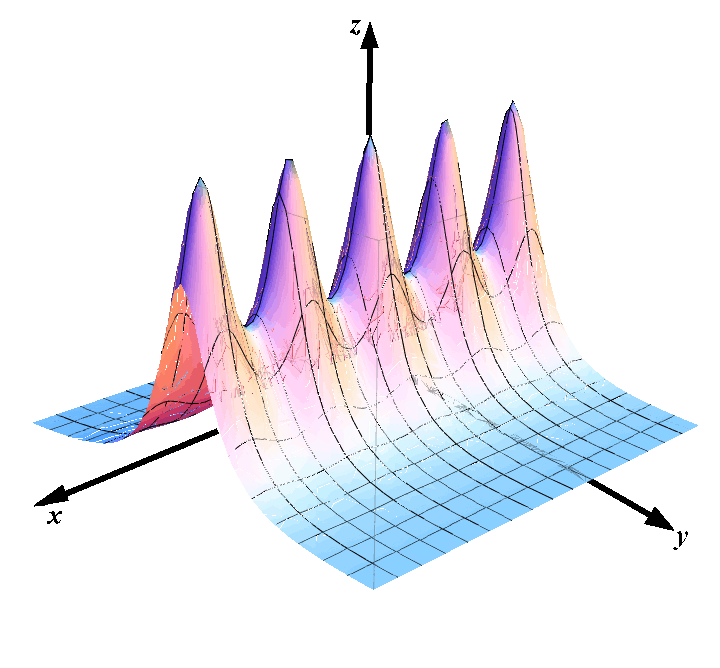## Level curves

The concept of level curves comes from geography where people use two-dimensional maps to draw the three dimensional terrain. This is done through a series of optical illusions. Geographers plot contour lines that connect points of the same altitude. These contours are called level curves. Then they use some colors, but we are not interested in that.

In mathematics, the concept of level curves has to be formally defined. We start by assuming that we are given a surface. We assume that the surface is defined as the graph of a function $$z=f(x,y)$$. For each constant $$c\in\mathbb R$$, the intersection of the plane $$z=1$$ with the surface $$z=f(x,y)$$ is a curve whose $$x$$ and $$y$$ coordinates satisfy the equation $$f(x,y)=c$$.

Level curve: Definition The level curve of the surface $$z=f(x,y)$$ corresponding to the level $$c\in\mathbb R$$ is defined as the curve in the $$xy$$ plane that satisfies the equation: $f(x,y)=c.$

For the function $$\displaystyle f(x,y)=\frac{9}{1+\sin^2 x+y^2}$$, the level sets are shown in the picture below.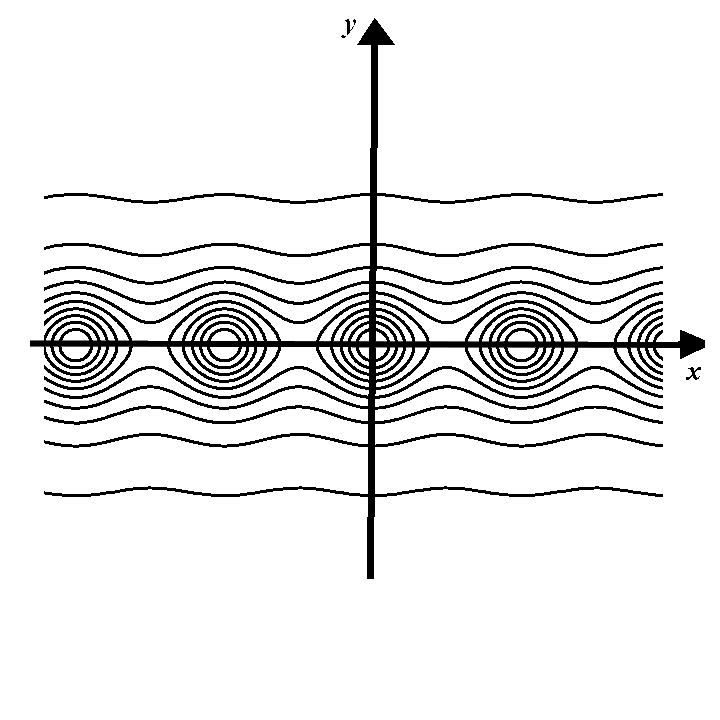## Practice problems

Problem 1. If $$f: \mathbb R^3\to\mathbb R^2$$ and $$g: \mathbb R^2\to\mathbb R$$, which of the following expressions is a well defined composition of functions?

(A) $$f(g(3,4), g(4,3))$$ $$\quad\quad$$ (B) $$f(g(-3,3))$$ $$\quad\quad$$ (C) $$g(f(3,5))$$ $$\quad\quad$$ (D) $$f(g(1,2),3,4)$$ $$\quad\quad$$ (E) $$g(f(9))$$

Problem 2. What is the largest possible domain of definition of the function $f(x,y)=\frac{x+y}{\sqrt{3x-9y^2}-3}?$
• (A) Empty set.
• (B) Entire $$\mathbb R^2$$.
• (C) All the points that do not belong to the parabolas $$x=3y^2$$ and $$x=3y^2+3$$.
• (D) All the points to the left of the parabola $$x=3y^2$$.
• (E) All the points to the right of the parabola $$x= 3y^2$$ that do not belong to the parabola $$x=3y^2+3$$.

Problem 3. Let $$\displaystyle f(x,y)=(x+y)\cdot \sin(-x^2-y^2)$$. Which of the pictures below represent the level curves of $$f$$?
• (A)• (B)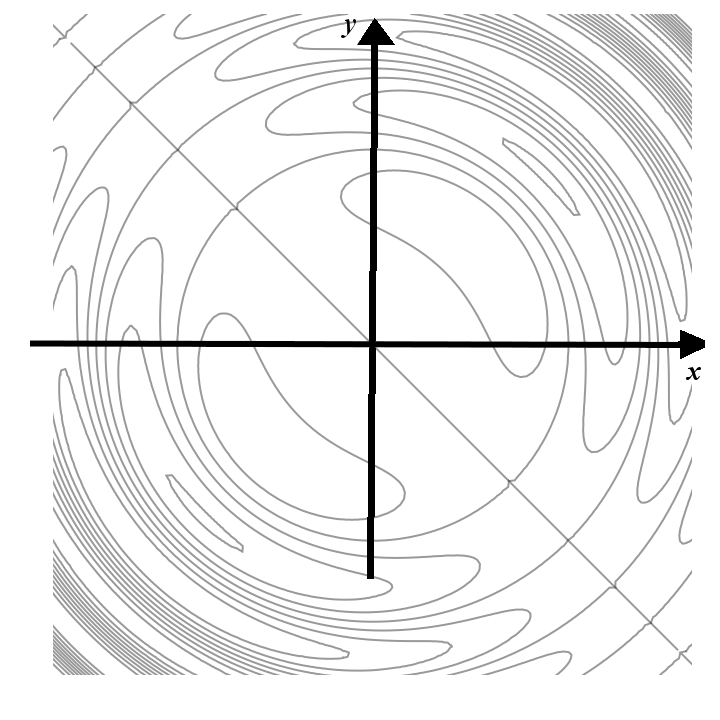• (C)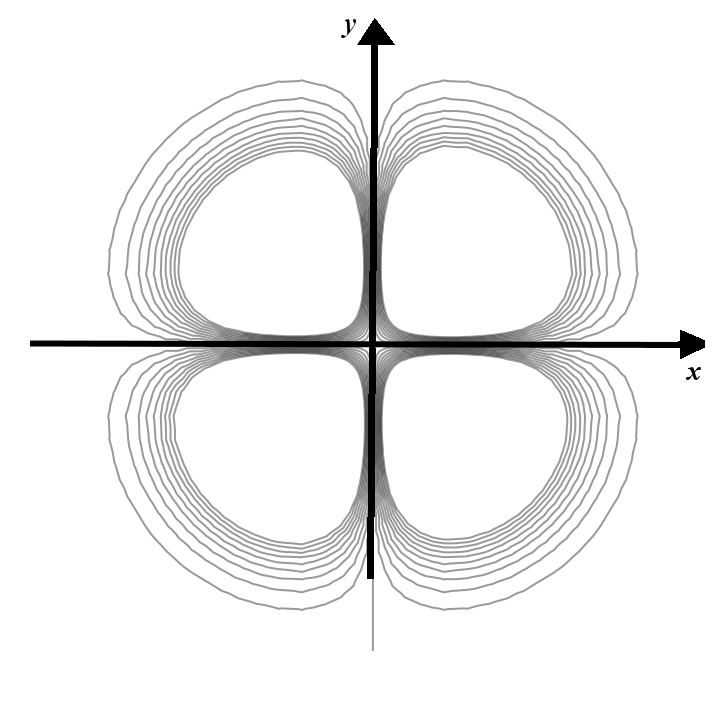• (D)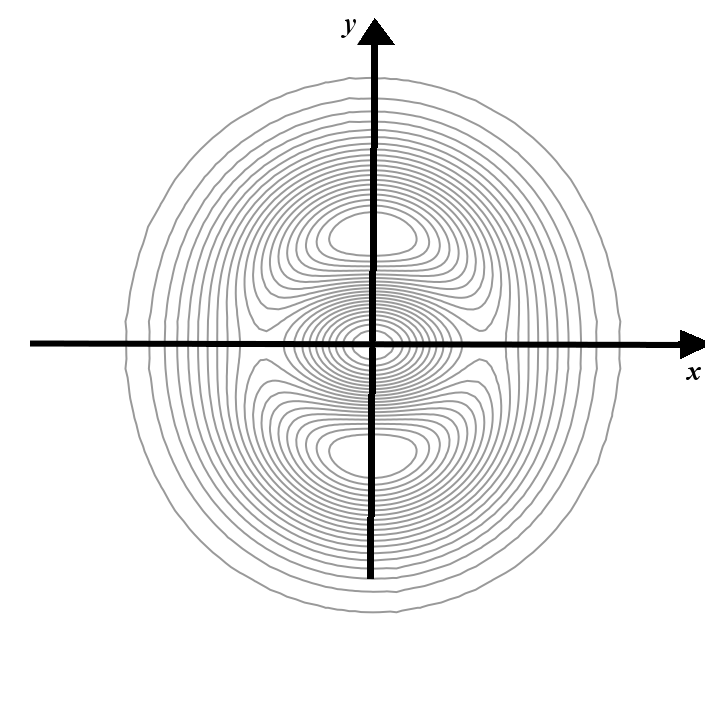• (E)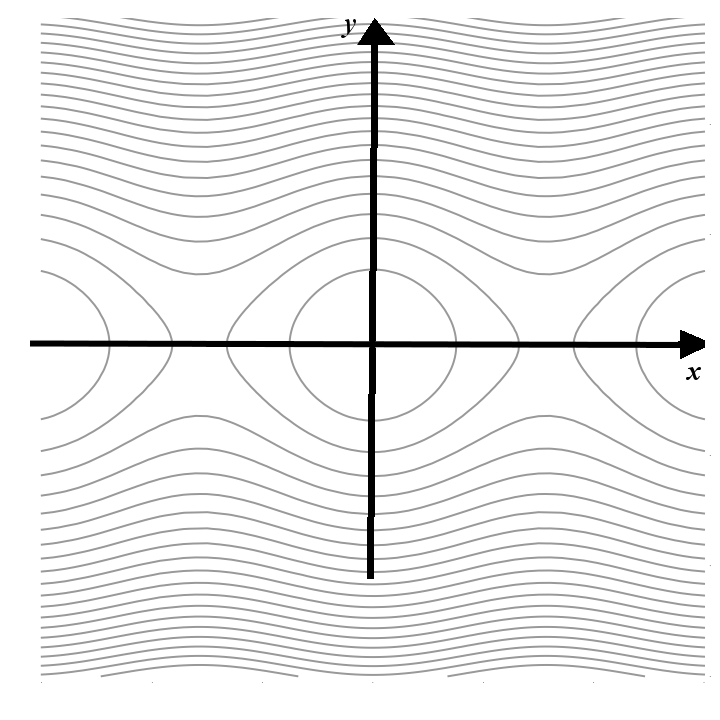Problem 4. If $$\displaystyle f(x,y)=3x^2-4y-3$$ and $$\displaystyle g(x)=5x-5$$, find $$g(f(7,6))$$.

Problem 5. If $$\displaystyle f(x,y)=3x-2y$$, $$g(x,y)=4x+5y$$, and $$h(x,y)=x\cdot y$$, evaluate $f\left( g(5,7), h(4,5)\right).$